MORE SITES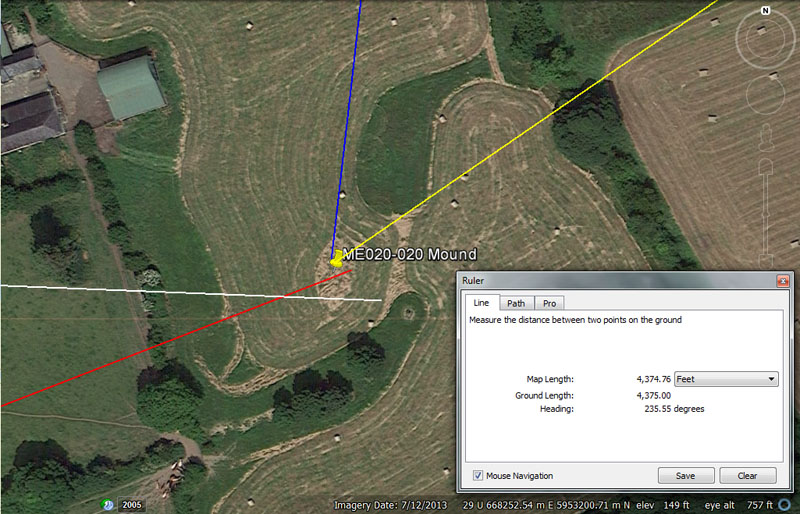Archaeologists are undecided about the significance of an oval mound at this position and whether or not it should have monument status. In consideration of the distance and angle codes inherent in the position, it generates multiple tutorials essential to students of navigation.

From Knowth (white line) the distance is 8750' @ 92.16-degrees (92 & 4/25ths).

A distance of 8750 is 1 & 2/3rds miles of 5250' each or 1.8 so-called Roman miles (using the overland foot of 11 & 2/3rds inches and pace of 58 & 1/3rd inches). The value of 8.75-days was very important to to the Sabbatical lunisolar calendar, which was allowed to run as 13-months of 28-days each (364-days per annum) for 7-years and thereby accruing 1.25-days of error per year. the correctional intercalary days were added during a 9-day (8.75) festival at the end of the seventh or Sabbatical Year and the count commenced anew.

The degree angle codes divisions of the 24883.2-mile equatorial circumference of which 92.16-miles = 1/270th part.

From Newgrange the distance is 5670' @ 68.75-degrees.

The 5670 value is both lunar and navigational coding simultaneously and 5670' equates to 5832 Roman feet of 11.66666" each or 1134 paces (alternatively, 5833.3333 X 11.664"). At the same time, there would be 12-periods of 567-days in the 6804-day lunar nutation cycle. likewise, there would be 45 periods of 56.7-days in the 2551.5-days (7.2 lunar years) tracked within the lunisolar Sabbatical calendar.

The angle of 68.75-degrees is navigational and there would be 68.75-miles in 1-degree of arc under the navigational method that configured the equatorial circumference as 24750-miles of 5280' each (130680000').

From Dowth mound the distance is 1260' @ 186.624-degrees.

This distance is, what later became, 2 Greek stadia (630') or 1200 Greek feet (12.6").

The angle of travel is in homage to the equatorial circumference of the Earth, thus 186624' would equate to 1/700th of the 130636800' equatorial circumference or, in miles, there would be 1 & 1/3rd X 18662.4-miles in the 24883.2-mile circumference of the world.

From Dowth Henge the distance is 4375' @ 55.55555-degrees.

This distance is navigational coding and there would be 4375' X 1.2 in a mile of 5250'. At the same time, there would be 1.11111 (1 & 1/9th) increments of 4375' in the so-called Roman mile of 4861.11111' (based upon an overland foot of 11 & 2/3rds inches). In other words, 4375' is .9 of that version or reading of a Roman overland mile.

This same tutorial, dealing with 1/9th fractions, seems to recur in the degree angle and there would be 87.5 X 55.55555' (55 & 5/9ths) in a Roman overland mile based upon the 11.66666" foot. Likewise, there would be 94.5 increments of 55.5555' in the 5250' mile.

As rudimentary or mundane as these mathematical exercises might seem to be, we must remember that the students of circa 3000 BC did not enjoy the luxury of endless supplies of paper or books, where all of the principles were recorded. Everything had to be committed to memory and the mind honed to work like a very efficient computer. Even in modern times writing paper has often been scarce or in short supply for students, and some post WWII children in Britain had to write their lessons in pencil, then rub out what they had written later after memorising the information. This way, the same blank page could be used many times over. Yet others had to write on slate tablets when paper was unobtainable, in short supply or considered to be a luxury beyond the budget of their parents.

With regards to this preoccupation of developing very acute memories, or what we might call photographic memories in modern times, Julius Caesar said the following about the late-era Druids of his day (the progeny of the much earlier teachers, continuing the ages-old tradition):

'They do not think it proper to commit these utterances to writing, although in all other matters and in their public and private accounts they make use of Greek characters. I believe that they have adopted the practice for two reasons- that they do not wish the rule to become common property, nor those who learn the rule to rely on writing and so neglect the cultivation of memory; and, in fact, it does usually happen that the assistance of writing tends to relax the diligence of the student and the action of memory...They also lecture on the stars in their motion, the magnitude of the Earth and its divisions, on natural history, on the power and government of God; and instruct the youth in these subjects' (see De Ballo Gallico, VII, 15, 16.).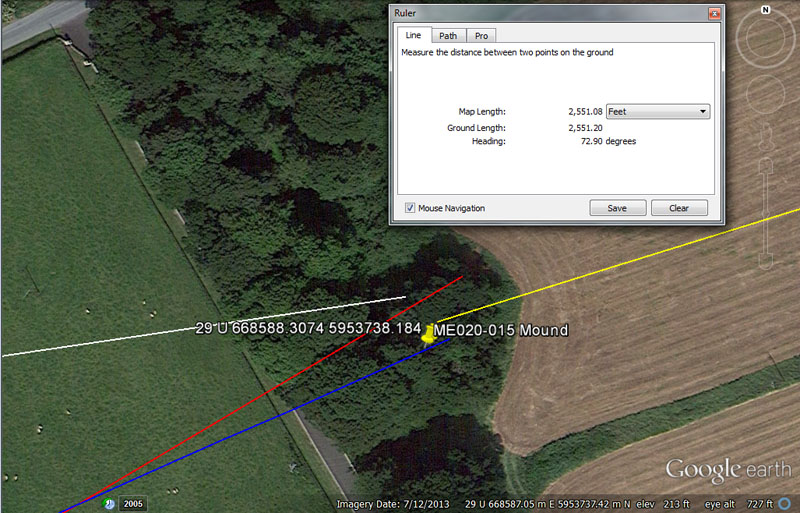The archaeological record places a mound at this position beneath the tree canopy.

From Knowth the distance is 9953.28' @ 81.675-degrees.

This is the same coded distance as the from the centre of Dowth Henge to the centre of Newgrange chambered mound and relates to the equatorial circumference of the Earth. Therefore, 9953.28-miles X 2.5 = 24883.2-miles or the 12 X 12 X 12 X 12 X 1.2-mile equatorial circumference.

The angle of 81.675 (81 & 27/40ths) is also equatorial circumference coding and the sum of 81675' (13.5-minutes of arc) would be 1/1600th of the 24750-mile (of 5280') circuit.

From Newgrange the distance is 7500' @ 59.4-degrees.

The 5250' mile is .7 of 7500'.

The angle of 59.4-degrees is navigational coding and relates to the mile of 5940' or 1-furlong greater than the 5280'-mile.

From Dowth Mound the distance is 1134' @ 66-degrees.

The value 1134 is a dynamic number which, in a mathematical progression, generates both navigational and lunar numbers. For example, the sum of 3-minutes of equatorial arc (18144') would be 1134' X 16. Likewise, 1134' would be 11.25 arc seconds (100.8') under the 24883.2-mile reading. The 6804-day lunar nutation cycle is 6 periods of 1134-days. The 2551.5-day lunar period monitored in the 7.2-lunar year and 7-solar year, lunisolar Sabbatical Calendar is 2.25 intervals of 1134-days.

The angle of 66-degrees is in homage to the furlong / furrowlong increment of 660' or the chain of 66', etc. There would be 25 furlongs in the ancient league of 16500' (3.125-miles) or 8 furlongs in a mile of 5280'.

From Dowth Henge the distance is 2551.5' @ 72.9-degrees.

This distance is in homage to the 2551.5-day lunar count (7.2 lunar years of 354.375-days each) within the Sabbatical Calendar system.

The angle of 72.9-degrees is also lunar coding, as well as navigational, and there would be 72.9 X 4.861111-days in a lunar year of 354.375-days (alternatively, 729.1111 X 4860). Depending upon the kind of calculation being done (lunar-period, volume, overland marching, etc.), the Roman foot seems to have been read as either 11.664" or 11.6666". The sum of 7.29" = 1/8th of a Roman pace of 58.32". The Bush Barrow Lozenge artefact (a memory device), which was found in the grave of a dignitary near Stonehenge, measures 7.29" for its longest cross-measure.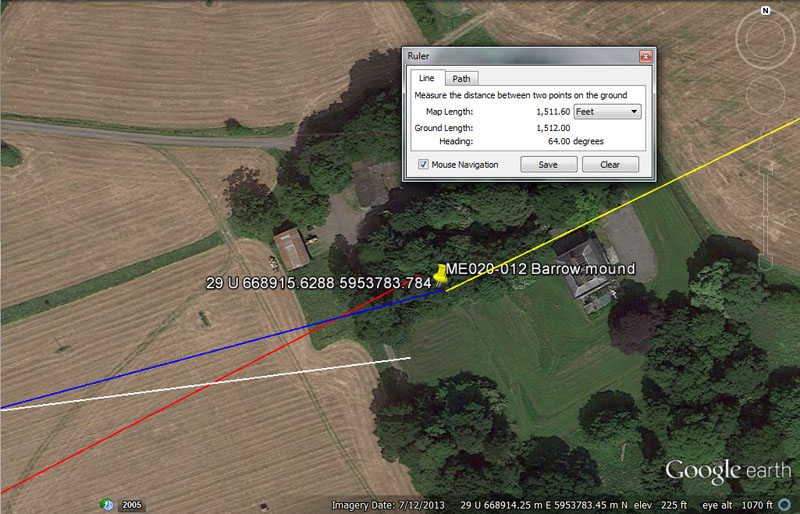A chambered mound sits under the tree canopy at this point.

From Knowth the distance is 11000' or 11025' @ either 262.5 (return) or 82.5-degrees.

It seems that from Knowth the preoccupation was to place navigating students equidistant between two chambered mound sites at a distance of 2/3rds of a league.

The degree angle to get resident students from Knowth chambered mound to these side-by-side chambered mounds at Dowth can be read as outwards running or return codes.

The sum of 11000' is 2/3rds of a league @ 16500', whereas 11025' would be 17.5 stadia of 630' or 2.1-miles of 5250'.

The 82.5-degrees reading is in homage to the ancient league of 16500' (8250' X 2). The 262.5 reading is in homage to the 5250' mile (2625' X 2).

From Newgrange the distance is 8400', which could also be read as 8437.5’ @ 62.5-degrees.

The value 8400' is 1.6-miles of 5250' or 1.25 of the ancient Irish miles (6720'). Also, 1-minute of equatorial arc (6048') = .72 of 8400'.

The value 84.375 (84 & 3/8ths) was much used in lunar calculations and there would be 4.2 intervals of 84.375-days (2024-hours) in a lunar year of 354.375-days. A mathematical progression based upon this value will provide many useful numbers used in lunar calculations.

The angle of 62.5-degrees, used in a mathematical progression, produces very useful numbers for navigation. The sum of 62.5' is 1/264th of the ancient league of 16500'.

From Dowth mound the distance is 2178’ @ 75-degrees.

This distance would be 1/60000th of the 24750-mile (of 5280') equatorial circumference (7920 leagues).

The degree angle is self explanatory, but one example would be that 75' X 70 = 5250', which became a Greek mile.

From Dowth Henge the distance is 1512’ @ 64-degrees.

Again, 1512' is 1/4th of 1-minute of equatorial arc (6048') or 15 arc seconds (100.8') and, at the same time, 1/100th the distance between Dowth Henge and the summit of Slieve Gullion Mountain's chambered cairn trig.

Again, 64 (8 X 8) is a multi-use value for all manner of lunar cycle or navigational calculations and recalls dividing up the 360-degree compass by half, quarter, eighth, sixteenth, thirty-second, sixty-fourth, etc. .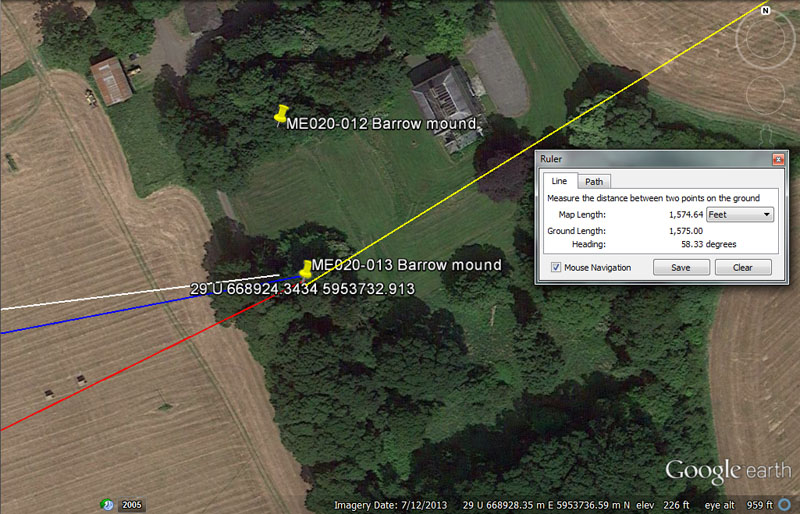Yet another chambered mound sits under the tree canopy at this point very close to Dowth Henge and, undoubtedly offered dormitory accommodation to students using the henge for cyclic-astronomical tutorials.

From Knowth = 11000' or 11025' @ 82.944-degrees.

Again 11000' is 2/3rds of an ancient league (16500') whereas 11025' would be 2.1-miles of 5250'.

The angle of 82.944-degrees (undoubtedly rounded to 83-degrees for travel but taught by the masters in pure numerical form as 82944) relates to the equatorial circumference of the Earth under the 24883.2-mile reading, of which 8294.4-miles would be 1/3rd part.

From Newgrange = 8400’ @ 63.7875-degrees (63 & 63/80ths).

Again, the value 8400' is 1.6-miles of 5250' or 1.25 of the ancient Irish miles (6720'). Also, 1-minute of equatorial arc (6048') = .72 of 8400'.

The degree angle is lunar and the 2551.5-days monitored in the lunisolar Sabbatical calendar is 4 periods of 637.875-days (637 & 7/8ths).

From Dowth Mound = 2160’ @ 259.2-degrees.

Both of these values relate to the 25920-year Precession of the Equinoxes, where the Sun spends 2160-years in each of the 12 houses of the zodiac. Also, 360-degrees is 21600-minutes of arc or 1296000 seconds of arc (half of 2592000).

From Dowth Henge = 1575’ @ 58.32-degrees.

The sum of 1575' would equate to 2.5 stadia of 630' each, whereas 15750' would be 3-miles of 5250'.

The degree angle is in homage to the Roman pace increment (58.32").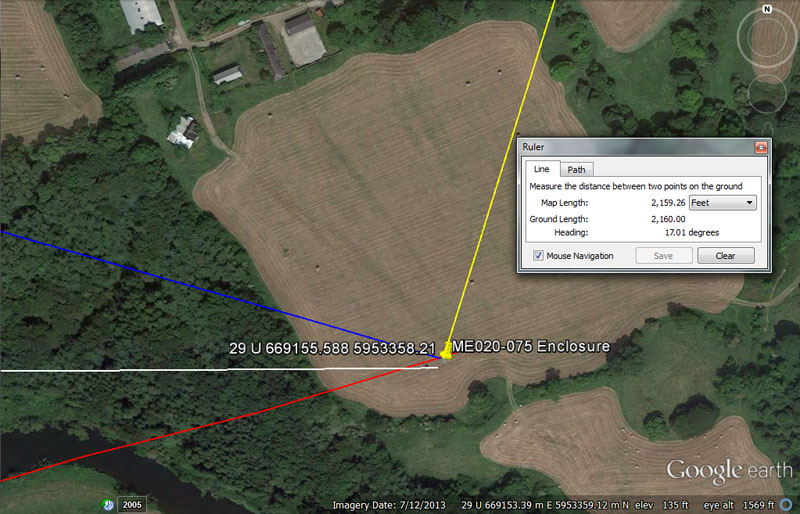The archaeological record places an enclosure at this position.

From Knowth = 11664’ @ 89.6-degrees.

This distance is what, at a much later epoch, became recognised as 2.4 Roman miles.

The angle of 89.6-degrees is navigational coding and the sum of 896' would be 1/75th of 67200' or 10 ancient Irish miles, whereas there would be 6.75 X 89.6' in 1-minute of equatorial arc (6048').

From Newgrange = 8640’ @ 73.3333-degrees.

The sum of 8640-years would be 1/3rd of the 25920-year Precession of the equinoxes cycle and 8640' would be 1/7th of 10-minutes of equatorial arc (60480'). The sun is 864,000-miles wide.

The degree angle is also navigational coding and 73.33333' (73 & 1/3rd) would be 1/72nd of a mile of 5280'.

From Dowth mound = 2592 @106.6666-degrees.

Again the 2592' value relates mnemonically to the 25920-year cycle of the Precession of the Equinoxes, where the date of the equinox needs to be adjusted by one day every 72-years in order to keep the calendar running 100% true. Likewise, a distance of 259.2-miles would be 1/96th of the 24883.2-mile equatorial circumference.

The angle of 106.6666-degrees is also navigational and 106.6666' is merely 1/3rd of 320'. The French once used an ancient foot measurement (Pied du Roi or Paris foot ... also used in Quebec), handed down from antiquity, of 1.06666' or 12.8".

From this foot, the ancient French had a Toise measurement of 6 such feet, equalling 6.4 feet of 12-inches. They also had a tradition called the "Lieue de 25 au degré" (25 Leagues per degree of the Earth's circumference). This league is said to have been the slightly drifted value of 2.764-miles, but would be perfect if read as 2.7648-miles. Thereby, 25 such leagues would be 69.12-miles (of 5280') for 1-degree of arc, culminating in a world of 24883.2-miles or 12 X 12 X 12 X 12 X 1.2.

From Dowth Henge = 2160’ @ 17.01-degrees return.

Again, the 2160 value relates to the Precession of the Equinoxes. The original, ancient bushel of England was 2160 cubic inches. Likewise, other civilisations shared the use of this number for their volume capacities and the Hebrew Desert Standard log for liquids was 21.6 cubic inches, while the Babylonian homer was 21600 cubic inches.

The degree angle relates to the 6804-day lunar nutation cycle, the quarter period of which was 1701-days.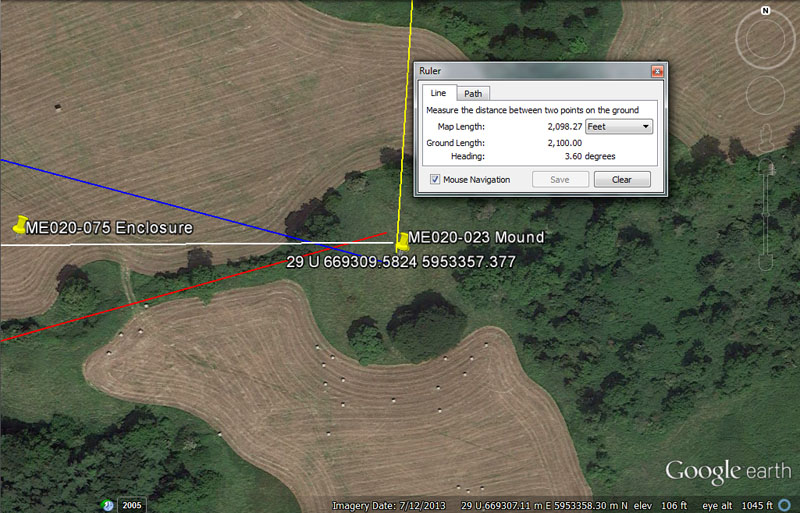A very impressive mound sits at this position.

From Knowth = 12175' @ 89.6-degrees.

The solar year @ 365.25-days is 3 X 121.75-days. The distance to the crown of the mound could also be read as 12150', which is strong lunar & navigational coding. This distance is 2.5 so-called Roman miles (12500 Roman feet using either the 11.664" lunar foot or 11.6666" overland foot). There would be 56 intervals of 121.5-days in the 6804-day lunar nutation cycle.

Again, the degree angle is navigational coding and there would be 75 X 89.6' in an ancient Irish mile.

From Newgrange = 9075’ @ 74.25-degrees.

The distance could be read simultaneously as either 9075' (1.5-minutes of equatorial arc under the 5280'-mile navigational system) or as 9072' (1.5-minutes of equatorial arc under the 5250'-mile navigational system).

The angle of 74.25-degrees is also navigational coding and relates to the ancient Scottish mile of 5940' (742.5' X 8 = 5940'). The distance of 7425-miles would be .3 of the 24750-mile equatorial circumference.

From Dowth Mound = 3456’ @ 105-degrees.

This distance is navigational coding and the sum of 345.6-miles would be 1/72nd of the 24883.2-mile equatorial circumference. Likewise, at Stonehenge there were 30 lintel stones lying horizontally around the Sarsen Circle ring, each measuring (or averaging) 11.52' on the external face. This equated to 345.6' of outer rim perimeter circuit, encoded for mnemonic recall of the equatorial circumference of the Earth.

The angle of 105-degrees is navigational coding and the Hebrew reed measurement was 10.5'. Likewise, 2-miles of 5250' = 10500'.

From Dowth Henge = 2100’ @ 3.6, 3.402 or  3.36-degrees return.

This distance is navigational coding and there would be 2.5 increments of 2100' in the 5250'-mile.

Several close-proximity codes, subjected to mathematical progression, could feature in tutorials related to the degree angle leading to this position, such as 3.6-degrees (compass), 3.402-degrees (lunar nutation cycle) or 3.36-degrees (diameter of the Earth).

ACROSS THE RIVER TO STALLEEN & OLDBRIDGE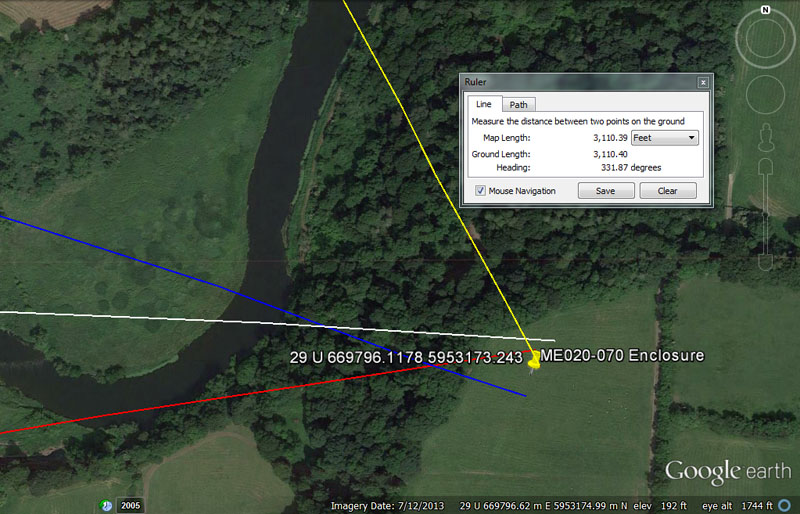The archaeological record places a giant enclosure at this position.

From Knowth = 13824’ @ 272.16-degrees.

This distance is navigational coding and there would be 1382.4-miles in a 1/18th segment (20-degrees of arc) of the 24883.2-mile equatorial circumference.

The degree angle is also navigational coding and a length of 27216' (4.5-minutes of arc) would be 1/4800th of the 24883.2-mile (of 5250') equatorial circumference.

From Newgrange = 10500’ @ 260 – 80-degrees.

The distance is 2-miles of 5250'.

This degree angle is the same as the one from Dowth Henge to Knowth chambered mound and return, providing useful values in both calendar and compass calculations, etc.

From Dowth Mound =  5156.25’ – 5184’ @ 288-degrees.

The distance of 5156.25' equates to 3000 Egyptian Royal cubits of 20.625" each. Also, 515.625-miles (515 & 5/8ths) would be 1/48th of the 24750-mile equatorial circumference or 7.5-degrees of arc.

If read as 5184', this is one of the most dynamic values of antiquity. The design slope angle of the Great Pyramid is 51.84-degrees. The area covered by the Great Pyramid @ 72 reeds (10.5') per side length is 5184 square reeds. The 25920-year cycle of Precession is 5 intervals of 5184-years.

As we proceed it will be demonstrated that the values 51562.5' and 51840' are encoded into structural positions on the Hill of Tara as they relate to Boyne River Valley structures.

The degree angle (288) is equally dynamic and 288' X 21 = 6048' (1-minute of equatorial arc) An ancient length increment dubbed the geomancer's mile was 14400' or half of 28800'. The Egyptian pyramid acre was 28800 square feet. The design diameter of the Aubrey Circle at Stonehenge is 288'.

From Dowth Henge = 3110.4’ @ 151.875-degrees.

A distance of 3110.4-miles would be 1/8th of the 24883.2-mile equatorial circumference.

The degree angle is a fraction of 4860 (1/32nd) and would have been useful in lunar cycle calculations.

Continue